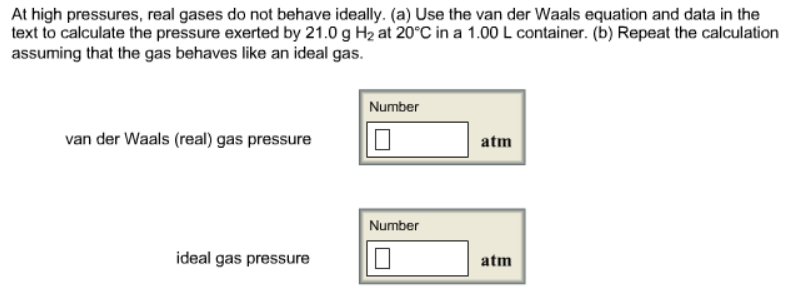# Problem: At high pressures, real gases do not behave ideally. (a) Use the van der Waals equation and data in the text to calculate the pressure exerted by 21.0 g H2 at 20°C in a 1.00 L container. (b) Repeat the calculation assuming that the gas behaves like an ideal gas.

###### FREE Expert Solution
95% (386 ratings)###### Problem Details

At high pressures, real gases do not behave ideally. (a) Use the van der Waals equation and data in the text to calculate the pressure exerted by 21.0 g H2 at 20°C in a 1.00 L container. (b) Repeat the calculation assuming that the gas behaves like an ideal gas.